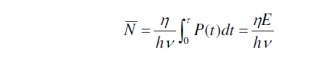Home | | Optical Communication and Networks | Fundamental Receiver Operation

## Chapter: Optical Communication and Networking : Fiber Optic Receiver and Measurements

1. Optical Receiver Operation 2. Error Sources

ü   Digital Signal Transmission

ü   Error Sources

ü   Probability of Error

ü   The Quantum Limit

Coherent Detection

### Digital Signal Transmissionü   A typical digital fiber transmission link is shown in Figure 4.1. The transmitted signal is a two-level binary data stream consisting of either a ‘0’ or a ‘1’ in a bit period Tb.

ü   The simplest technique for sending binary data is amplitude-shift keying, wherein a voltage level is switched between on or off values.

ü   The resultant signal wave thus consists of a voltage pulse of amplitude V when a binary 1 occurs and a zero-voltage-level space when a binary 0 occurs.

ü   An electric current i(t) can be used to modulate directly an optical source to produce an optical output power P(t).

ü   In the optical signal emerging from the transmitter, a ‘1’ is represented by a light pulse of duration Tb,whereas a ‘0’ is the absence of any light.

ü   The optical signal that gets coupled from the light source to the fiber becomes attenuated and distorted as it propagates along the fiber waveguide. Upon reaching the receiver, either a PIN or an APD converts the optical signal back to an electrical format.

ü   A decision circuit compares the amplified signal in each time slot with a threshold level.

ü   If the received signal level is greater than the threshold level, a ‘1’ is said to have been received.

ü   If the voltage is below the threshold level, a ‘0’ is assumed to have been received.

## 2. Error Sources

ü   Errors in the detection mechanism can arise from various noises and disturbances associates with the signal detection system.

ü   The two most common samples of the spontaneous fluctuations are shot noise and thermal noise.

ü   Shot noise arises in electronic devices because of the discrete nature of current flow in the device.

ü   Thermal noise arises from the random motion of electrons in a conductor.

ü   The random arrival rate of signal photons produces a quantum (or shot) noise at the photodetector. This noise depends on the signal level.

ü   This noise is of particular importance for PIN receivers that have large optical input levels and for APD receivers.

ü   When using an APD, an additional shot noise arises from the statistical nature of the multiplication process. This noise level increases with increasing avalanche gain M.ü   Thermal noises arising from the detector load resistor and from the amplifier electronics tend to dominate in applications with low SNR when a PIN photodiode is used.

ü   When an APD is used in low-optical-signallevel applications, the optimum avalanche gain is determined by a design tradeoff between the thermal noise and the gain-dependent quantum noise.

ü   The primary photocurrent generated by the photodiode is a time-varying Poisson process.

ü   If the detector is illuminated by an optical signal P(t), then the average number of electron-hole pairs generated in a time t iswhere h is the detector quantum efficiency, hn is the photon energy, and E is the energy received in a time interval .

ü   The actual number of electron-hole pairs n that are generated fluctuates from the average according to the Poisson distributionwhere Pr(n) is the probability that n electrons are emitted in an interval tü   For a detector with a mean avalanche gain M and an ionization rate ratio k, the excess noise factor F(M) for electron injection iswhere  the factor x ranges between 0 and 1.0 depending on the photodiode material.

ü   A further error source is attributed to intersymbol interference (ISI), which results from pulse spreading in the optical fiber.

ü   The fraction of energy remaining in the appropriate time slot is designated by g, so that 1-g is the fraction of energy that has spread into adjacent time slots.

Study Material, Lecturing Notes, Assignment, Reference, Wiki description explanation, brief detail
Optical Communication and Networking : Fiber Optic Receiver and Measurements : Fundamental Receiver Operation |